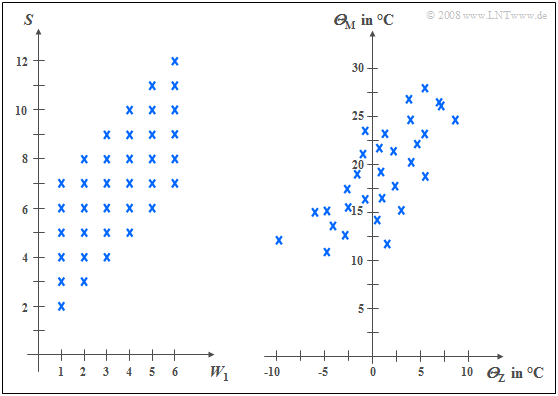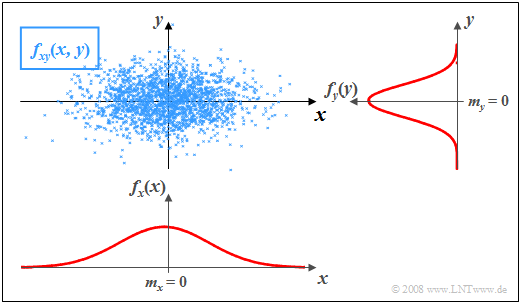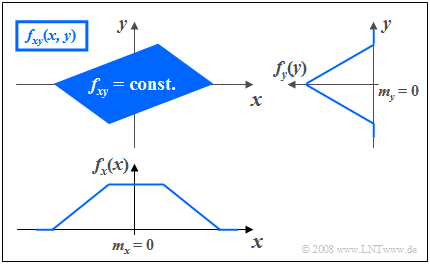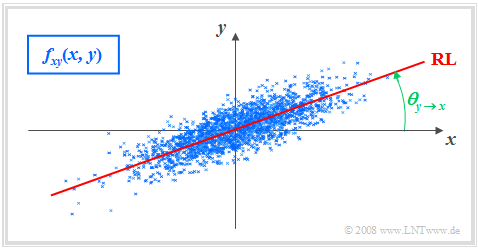# Two-Dimensional Random Variables

## # OVERVIEW OF THE FOURTH MAIN CHAPTER #

Now random variables with statistical bindings are treated and illustrated by typical examples.

After the general description of two-dimensional random variables,  we turn to

1. the  "auto-correlation function",
2. the  "cross-correlation function"
3. and the associated spectral functions  $($"power-spectral density",  "cross power-spectral density"$)$.

Specifically,  this chapter covers:

• the statistical description of  »two-dimensional random variables«  using the  »joint PDF«,
• the difference between  »statistical dependence«  and  »correlation«,
• the classification features  »stationarity«  and  »ergodicity«  of stochastic processes,
• the definitions of  »auto-correlation function«  $\rm (ACF)$  and  »power-spectral density«  $\rm (PSD)$,
• the definitions of  »cross-correlation function«  $\rm (CCF)$   and  »cross power-spectral density«  $\rm (C–PSD)$,
• the numerical determination of all these variables in the two- and multi-dimensional case.

## Properties and examples

As a transition to the  $\text{correlation functions}$  we now consider two random variables  $x$  and  $y$,  between which statistical dependences exist.

Each of these two random variables can be described on its own with the introduced characteristic variables corresponding

$\text{Definition:}$  To describe the statistical dependences between two variables  $x$  and  $y$,  it is convenient to combine the two components
into one   »two-dimensional random variable«   or   »2D random variable«  $(x, y)$.

• The individual components can be signals such as the real and imaginary parts of a phase modulated signal.
• But there are a variety of two-dimensional random variables in other domains as well,  as the following example will show.

$\text{Example 1:}$  The left diagram is from the random experiment  "Throwing two dice".Two examples of statistically dependent random variables
• Plotted to the right is the number of the first die  $(W_1)$,
• plotted to the top is the sum  $S$  of both dice.

The two components here are each discrete random variables between which there are statistical dependencies:

• If  $W_1 = 1$,  then the sum  $S$  can only take values between  $2$  and  $7$,  each with equal probability.
• In contrast,  for  $W_1 = 6$  all values between  $7$  and  $12$  are possible,  also with equal probability.

In the right diagram,  the maximum temperatures of the  $31$ days in May 2002 of Munich  (to the top)  and the mountain  "Zugspitze"  (to the right)  are contrasted.  Both random variables are continuous in value:

• Although the measurement points are about  $\text{100 km}$  apart,  and on the Zugspitze,  it is on average about   $20$  degrees colder than in Munich due to the different altitudes  $($nearly  $3000$  versus  $520$  meters$)$,  one recognizes nevertheless a certain statistical dependence between the two random variables  ${\it Θ}_{\rm M}$  and  ${\it Θ}_{\rm Z}$.
• If it is warm in Munich,  then pleasant temperatures are also more likely to be expected on the Zugspitze.  However,  the relationship is not deterministic:  The coldest day in May 2002 was a different day in Munich than the coldest day on the Zugspitze.

## Joint probability density function

We restrict ourselves here mostly to continuous valued random variables.

• However,  sometimes the peculiarities of two-dimensional discrete random variables are discussed in more detail.
• Most of the characteristics previously defined for one-dimensional random variables can be easily extended to two-dimensional variables.

$\text{Definition:}$  The  probability density function  $\rm (PDF)$  of the two-dimensional random variable at the location  $(x_\mu,\hspace{0.1cm} y_\mu)$   ⇒   »joint PDF«   or   »2D–PDF«
is an extension of the one-dimensional PDF  $(∩$  denotes logical  "and"  operation$)$:

$$f_{xy}(x_\mu, \hspace{0.1cm}y_\mu) = \lim_{\left.{\Delta x\rightarrow 0 \atop {\Delta y\rightarrow 0} }\right.}\frac{ {\rm Pr}\big [ (x_\mu - {\rm \Delta} x/{\rm 2} \le x \le x_\mu + {\rm \Delta} x/{\rm 2}) \cap (y_\mu - {\rm \Delta} y/{\rm 2} \le y \le y_\mu +{\rm \Delta}y/{\rm 2}) \big] }{ {\rm \Delta} \ x\cdot{\rm \Delta} y}.$$

$\rm Note$:

• If the two-dimensional random variable is discrete,  the definition must be slightly modified:
• For the lower range limits,  the  "less-than-equal"  sign must then be replaced by  "less-than"  according to the section  "CDF for discrete-valued random variables"

Using this joint PDF $f_{xy}(x, y)$,  statistical dependencies within the two-dimensional random variable  $(x,\ y)$  are also fully captured in contrast to the two one-dimensional density functions   ⇒   »marginal probability density functions«   $($or   "edge probability density functions"$)$:

$$f_{x}(x) = \int _{-\infty}^{+\infty} f_{xy}(x,y) \,\,{\rm d}y ,$$
$$f_{y}(y) = \int_{-\infty}^{+\infty} f_{xy}(x,y) \,\,{\rm d}x .$$

These two marginal probability density functions  $f_x(x)$  and  $f_y(y)$

• provide only statistical information about the individual components  $x$  and  $y$, resp.
• but not about the statistical bindings between them.

## Two-dimensional cumulative distribution function

$\text{Definition:}$  Like the  "2D–PDF",  the  »2D cumulative distribution function«  is merely a useful extension of the  $\text{one-dimensional distribution function}$  $\rm (CDF)$:

$$F_{xy}(r_{x},r_{y}) = {\rm Pr}\big [(x \le r_{x}) \cap (y \le r_{y}) \big ] .$$

The following similarities and differences between the  "1D–CDF"  and the  2D–CDF"  emerge:

• The functional relationship between two-dimensional PDF and two-dimensional CDF is given by integration as in the one-dimensional case,  but now in two dimensions.  For continuous valued random variables:
$$F_{xy}(r_{x},r_{y})=\int_{-\infty}^{r_{y}} \int_{-\infty}^{r_{x}} f_{xy}(x,y) \,\,{\rm d}x \,\, {\rm d}y .$$
• Inversely,  the probability density function can be given from the cumulative distribution function by partial differentiation to  $r_{x}$  and  $r_{y}$:
$$f_{xy}(x,y)=\frac{{\rm d}^{\rm 2} F_{xy}(r_{x},r_{y})}{{\rm d} r_{x} \,\, {\rm d} r_{y}}\Bigg|_{\left.{r_{x}=x \atop {r_{y}=y}}\right.}.$$
• Relative to the two-dimensional cumulative distribution function  $F_{xy}(r_{x}, r_{y})$  the following limits apply:
$$F_{xy}(-\infty,-\infty) = 0,$$
$$F_{xy}(r_{\rm x},+\infty)=F_{x}(r_{x} ),$$
$$F_{xy}(+\infty,r_{y})=F_{y}(r_{y} ) ,$$
$$F_{xy} (+\infty,+\infty) = 1.$$
• From the last equation  $($infinitely large  $r_{x}$  and  $r_{y})$  we obtain the  »normalization condition«  for the  "2D– PDF":
$$\int_{-\infty}^{+\infty} \int_{-\infty}^{+\infty} f_{xy}(x,y) \,\,{\rm d}x \,\,{\rm d}y=1 .$$

$\text{Conclusion:}$  Note the significant difference between one-dimensional and two-dimensional random variables:

• For one-dimensional random variables,  the area under the PDF always yields the value  $1$.
• For two-dimensional random variables,  the PDF volume is always equal to  $1$.

## PDF for statistically independent components

For statistically independent components  $x$,  $y$  the following holds for the joint probability according to the elementary laws of statistics if  $x$  and  $y$  are continuous in value:

$${\rm Pr} \big[(x_{\rm 1}\le x \le x_{\rm 2}) \cap( y_{\rm 1}\le y\le y_{\rm 2})\big] ={\rm Pr} (x_{\rm 1}\le x \le x_{\rm 2}) \cdot {\rm Pr}(y_{\rm 1}\le y\le y_{\rm 2}) .$$

For this,  in the case of independent components can also be written:

$${\rm Pr} \big[(x_{\rm 1}\le x \le x_{\rm 2}) \cap(y_{\rm 1}\le y\le y_{\rm 2})\big] =\int _{x_{\rm 1}}^{x_{\rm 2}}f_{x}(x) \,{\rm d}x\cdot \int_{y_{\rm 1}}^{y_{\rm 2}} f_{y}(y) \, {\rm d}y.$$

$\text{Definition:}$  It follows that for  »statistical independence«  the following condition must be satisfied with respect to the  »two-dimensional probability density function«:

$$f_{xy}(x,y)=f_{x}(x) \cdot f_y(y) .$$

$\text{Example 2:}$  In the graph,  the instantaneous values of a two-dimensional random variable are plotted as points in the  $(x,\, y)$–plane.

• Ranges with many points,  which accordingly appear dark,  indicate large values of the two-dimensional PDF  $f_{xy}(x,\, y)$.
• In contrast,  the random variable  $(x,\, y)$  has relatively few components in rather bright areas.Statistically independent components:  $f_{xy}(x, y)$, $f_{x}(x)$  and $f_{y}(y)$

The graph can be interpreted as follows:

• The marginal probability densities  $f_{x}(x)$  and  $f_{y}(y)$  already indicate that both  $x$  and  $y$  are Gaussian and zero mean,  and that the random variable  $x$  has a larger standard deviation than  $y$.
• $f_{x}(x)$  and  $f_{y}(y)$  do not provide information on whether or not statistical bindings exist for the random variable  $(x,\, y)$.
• However,  using the  "2D-PDF"  $f_{xy}(x,\, y)$  one can see that here there are no statistical bindings between the two components  $x$  and  $y$.
• With statistical independence,  any cut through  $f_{xy}(x, y)$  parallel to  $y$–axis yields a function that is equal in shape to the marginal PDF  $f_{y}(y)$.  Similarly,  all cuts parallel to  $x$–axis are equal in shape to  $f_{x}(x)$.
• This fact is equivalent to saying that in this example  $f_{xy}(x,\, y)$  can be represented as the product of the two marginal probability densities:
$$f_{xy}(x,\, y)=f_{x}(x) \cdot f_y(y) .$$

## PDF for statistically dependent components

If there are statistical bindings between  $x$  and  $y$,  then different cuts parallel to  $x$– and  $y$–axis,  resp.,  yield different  (non-shape equivalent)  functions.  In this case,  of course,  the joint PDF cannot be described as a product of the two  (one-dimensional)  marginal probability densities functions either.

$\text{Example 3:}$  The graph shows the instantaneous values of a two-dimensional random variable in the  $(x, y)$–plane.Statistically dependent components:  $f_{xy}(x, y)$, $f_{x}(x)$,  $f_{y}(y)$

Now,  unlike  $\text{Example 2}$  there are statistical bindings between  $x$  and  $y$.

• The two-dimensional random variable takes all  "2D" values with equal probability in the parallelogram drawn in blue.
• No values are possible outside the parallelogram.

One recognizes from this representation:

1. Integration over $f_{xy}(x, y)$  parallel to the  $x$–axis leads to the triangular marginal PDF  $f_{y}(y)$,  integration parallel to  $y$–axis to the trapezoidal PDF $f_{x}(x)$.
2. From the joint PDF $f_{xy}(x, y)$  it can already be guessed that for each  $x$–value on statistical average, a different  $y$–value is to be expected.
3. This means that the components  $x$  and  $y$  are statistically dependent on each other.

## Expected values of two-dimensional random variables

A special case of statistical dependence is  "correlation".

$\text{Definition:}$  Under  »correlation«  one understands a  "linear dependence"  between the individual components  $x$  and  $y$.

• Correlated random variables are thus always also statistically dependent.
• But not every statistical dependence implies correlation at the same time.

To quantitatively capture correlation,  one uses various expected values of the two-dimensional random variable  $(x, y)$.

These are defined analogously to the one-dimensional case,

• according to  "Chapter 2"  (for discrete valued random variables).
• and  "Chapter 3"  (for continuous valued random variables):

$\text{Definition:}$  For the  (non-centered)  »moments«  the following relation holds:

$$m_{kl}={\rm E}\big[x^k\cdot y^l\big]=\int_{-\infty}^{+\infty}\hspace{0.2cm}\int_{-\infty}^{+\infty} x\hspace{0.05cm}^{k} \cdot y\hspace{0.05cm}^{l} \cdot f_{xy}(x,y) \, {\rm d}x\, {\rm d}y.$$

Thus,  the two linear means are  $m_x = m_{10}$  and  $m_y = m_{01}.$

$\text{Definition:}$  The  »central moments«  $($related to  $m_x$  and  $m_y)$  are:

$$\mu_{kl} = {\rm E}\big[(x-m_{x})\hspace{0.05cm}^k \cdot (y-m_{y})\hspace{0.05cm}^l\big] .$$

In this general definition equation,  the variances  $σ_x^2$  and  $σ_y^2$  of the two individual components are included by  $\mu_{20}$  and  $\mu_{02}$,  resp.

$\text{Definition:}$  Of particular importance is the  »covariance«  $(k = l = 1)$,  which is a measure of the  "linear statistical dependence"  between the variables  $x$  and  $y$:

$$\mu_{11} = {\rm E}\big[(x-m_{x})\cdot(y-m_{y})\big] = \int_{-\infty}^{+\infty} \int_{-\infty}^{+\infty} (x-m_{x}) \cdot (y-m_{y})\cdot f_{xy}(x,y) \,{\rm d}x \, {\rm d}y .$$

In the following,  we also denote the covariance  $\mu_{11}$  in part by  "$\mu_{xy}$",  if the covariance refers to the random variables  $x$  and  $y$.

Notes:

• The covariance  $\mu_{11}=\mu_{xy}$  is related to the non-centered moment  $m_{11} = m_{xy} = {\rm E}\big[x \cdot y\big]$  as follows:
$$\mu_{xy} = m_{xy} -m_{x }\cdot m_{y}.$$
• This equation is enormously advantageous for numerical evaluations,  since  $m_{xy}$,  $m_x$  and  $m_y$  can be found from the sequences  $〈x_v〉$  and  $〈y_v〉$  in a single run.
• On the other hand,  if one were to calculate the covariance  $\mu_{xy}$  according to the above definition equation,  one would have to find the mean values  $m_x$  and  $m_y$  in a first run and could then only calculate the expected value  ${\rm E}\big[(x - m_x) \cdot (y - m_y)\big]$  in a second run.

$\text{Example 4:}$  In the first two rows of the table,  the first elements of two random sequences  $〈x_ν〉$  and  $〈y_ν〉$  are entered.  In the last row, the respective products  $x_ν - y_ν$  are given.

• By averaging over ten sequence elements in each case,  one obtains
$$m_x =0.5,\ \ m_y = 1, \ \ m_{xy} = 0.69.$$
• This directly results in the value for the covariance:
$$\mu_{xy} = 0.69 - 0.5 · 1 = 0.19.$$

Without knowledge of the equation  $\mu_{xy} = m_{xy} - m_x\cdot m_y$  one would have had to first determine the means  $m_x$  and  $m_y$  in the first run,  and then determine the covariance  $\mu_{xy}$  as the expected value of the product of the zero mean variables in a second run.

## Correlation coefficient

With statistical independence of the two components  $x$  and  $y$   the covariance  $\mu_{xy} \equiv 0$.  This case has already been considered in  $\text{Example 2}$  in the section  "PDF for statistically independent components".

• But the result  $\mu_{xy} = 0$  is also possible for statistically dependent components  $x$  and  $y$  namely when they are uncorrelated,  i.e.  "linearly independent".
• The statistical dependence is then not of first order,  but of higher order,  for example corresponding to the equation  $y=x^2.$

One speaks of  »complete correlation«  when the  (deterministic)  dependence between  $x$  and  $y$  is expressed by the equation  $y = K · x$.  Then the covariance is given by:

• $\mu_{xy} = σ_x · σ_y$  with positive  $K$  value,
• $\mu_{xy} = - σ_x · σ_y$  with negative  $K$  value.

Therefore,  instead of the  "covariance"  one often uses the so-called  "correlation coefficient"  as descriptive quantity.

$\text{Definition:}$  The  »correlation coefficient«  is the quotient of the covariance  $\mu_{xy}$  and the product of the standard deviations  $σ_x$  and  $σ_y$  of the two components:

$$\rho_{xy}=\frac{\mu_{xy} }{\sigma_x \cdot \sigma_y}.$$

The correlation coefficient  $\rho_{xy}$  has the following properties:

• Because of normalization,   $-1 \le ρ_{xy} ≤ +1$  always holds.
• If the two random variables  $x$  and  $y$  are uncorrelated,  then  $ρ_{xy} = 0$.
• For strict linear dependence between  $x$  and  $y$   ⇒   $ρ_{xy}= ±1$   ⇒   complete correlation.
• A positive correlation coefficient means that when  $x$  is larger,  on statistical average,  $y$  is also larger than when  $x$  is smaller.
• In contrast,  a negative correlation coefficient expresses that  $y$  becomes smaller on average as  $x$  increases.

$\text{Example 5:}$  The following conditions apply:

1. The considered components  $x$  and  $y$  each have a Gaussian PDF.
2. The two standard deviations are different  $(σ_y < σ_x)$.
3. The correlation coefficient is  $ρ_{xy} = 0.8$.

Unlike  $\text{Example 2}$  with statistically independent components   ⇒   $ρ_{xy} = 0$  $($even though  $σ_y < σ_x)$  one recognizes that here

• with larger  $x$–value, on statistical average,  $y$  is also larger
• than with a smaller  $x$–value.

## Regression line

$\text{Definition:}$  The  »regression line«  – sometimes called  "correlation line" –  is the straight line  $y = K(x)$  in the  $(x, y)$–plane through the  "midpoint"  $(m_x, m_y)$.Two-dimensional Gaussian PDF with regression line  $\rm (RL)$

The regression line has the following properties:

• The mean square deviation from this straight line  - viewed in  $y$–direction and averaged over all  $N$  points -  is minimal:
$$\overline{\varepsilon_y^{\rm 2} }=\frac{\rm 1}{N} \cdot \sum_{\nu=\rm 1}^{N}\; \;\big [y_\nu - K(x_{\nu})\big ]^{\rm 2}={\rm minimum}.$$
• The regression line can be interpreted as a kind of  "statistical symmetry axis".  The equation of the straight line is:
$$y=K(x)=\frac{\sigma_y}{\sigma_x}\cdot\rho_{xy}\cdot(x - m_x)+m_y.$$
• The angle taken by the regression line to the  $x$–axis is:
$$\theta_{y\hspace{0.05cm}\rightarrow \hspace{0.05cm}x}={\rm arctan}\ (\frac{\sigma_{y} }{\sigma_{x} }\cdot \rho_{xy}).$$

By this nomenclature it should be made clear that we are dealing here with the regression of  $y$  on  $x$.

• The regression in the opposite direction  – that is, from  $x$  to  $y$ –  on the other hand,  means the minimization of the mean square deviation in  $x$–direction.
• The  (German language)  applet  "Korrelation und Regressionsgerade"   ⇒   "Correlation Coefficient and Regression Line"  illustrates
that in general  $($if  $σ_y \ne σ_x)$  for the regression of  $x$  on  $y$  will result in a different angle and thus a different regression line:
$$\theta_{x\hspace{0.05cm}\rightarrow \hspace{0.05cm} y}={\rm arctan}\ (\frac{\sigma_{x}}{\sigma_{y}}\cdot \rho_{xy}).$$